### Home > CALC > Chapter 3 > Lesson 3.2.2 > Problem3-60

3-60.
1. Differentiate the following expressions. Homework Help ✎

1.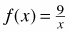2. f(x) = (−3x7 − 6x)

3. f(x) = (5x−4 )

4. f(x) = m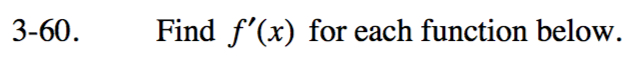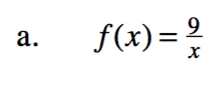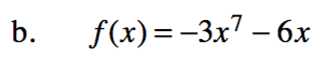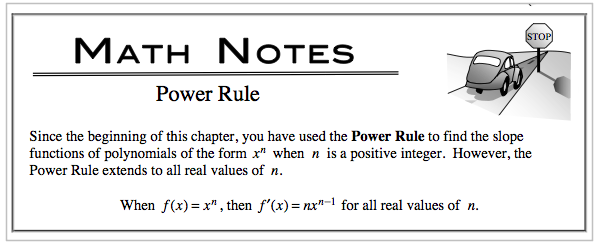f '(x) = −21x6 − 6

Refer to hint in part (b).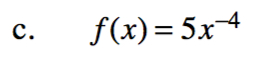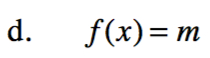m is a constant. What is the slope of the a
horizontal line y = m?